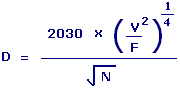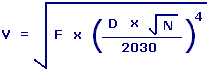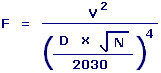Subwoofer Vent Minimum Port Diameter Calculators Enter value, select unit and click on calculate. Result will be displayed.

Calculate Minimum Port Vent Diameter
Calculate Maximum Air Volume
Calculate Tuning Frequency

 Web www.calculatoredge.com

Calculate Minimum Port Vent DiameterD = Minimum Port Vent Diameter V = Maximum Air Volume F = Tuning Frequency N = Number of Ports
 Enter your values: Maximum Air Volume: Meter^3 Centimeter^3 Foot^3 Inch^3 Kilometer^3 Yard^3 Liter Milliliter Deciliter Cord Firewood Cord Foot Wood Gallon UK Gallon US Liquid Ounce UK Fluid Ounce US Fluid Quart US Tuning Frequency: Hertz 1 / Second Cycle / Second Rotation / Second Number of Ports: Result: Minimum Port Vent Diameter: Centimeter

Calculate Maximum Air VolumeV = Maximum Air Volume F = Tuning Frequency D = Minimum Port Vent Diameter N = Number of Ports
 Enter your values: Tuning Frequency: Hertz 1 / Second Cycle / Second Rotation / Second Minimum Vent Port Diameter: Centimeter Meter Attometer Dekameter Decimeter Exameter Femtometer Foot Hectometer Inch Kilometer Megameter Micrometer Mile Millimeter Nanometer Petameter Picometer Yard Number of Ports or Vents: Result: Maximum Air Volume: Meter3

Calculate Tuning FrequencyF = Tuning Frequency V = Maximum Air Volume D = Minimum Port Vent Diameter N = Number of Ports
 Enter your values: Maximum Air Volume: Meter^3 Centimeter^3 Foot^3 Inch^3 Kilometer^3 Yard^3 Liter Milliliter Deciliter Cord Firewood Cord Foot Wood Gallon UK Gallon US Liquid Ounce UK Fluid Ounce US Fluid Quart US Minimum Vent Port Diameter: Centimeter Meter Attometer Dekameter Decimeter Exameter Femtometer Foot Hectometer Inch Kilometer Megameter Micrometer Mile Millimeter Nanometer Petameter Picometer Yard Number of Ports or Vents: Result: Tuning Frequency: HertzLINKSDISCLAIMERCONTACT US